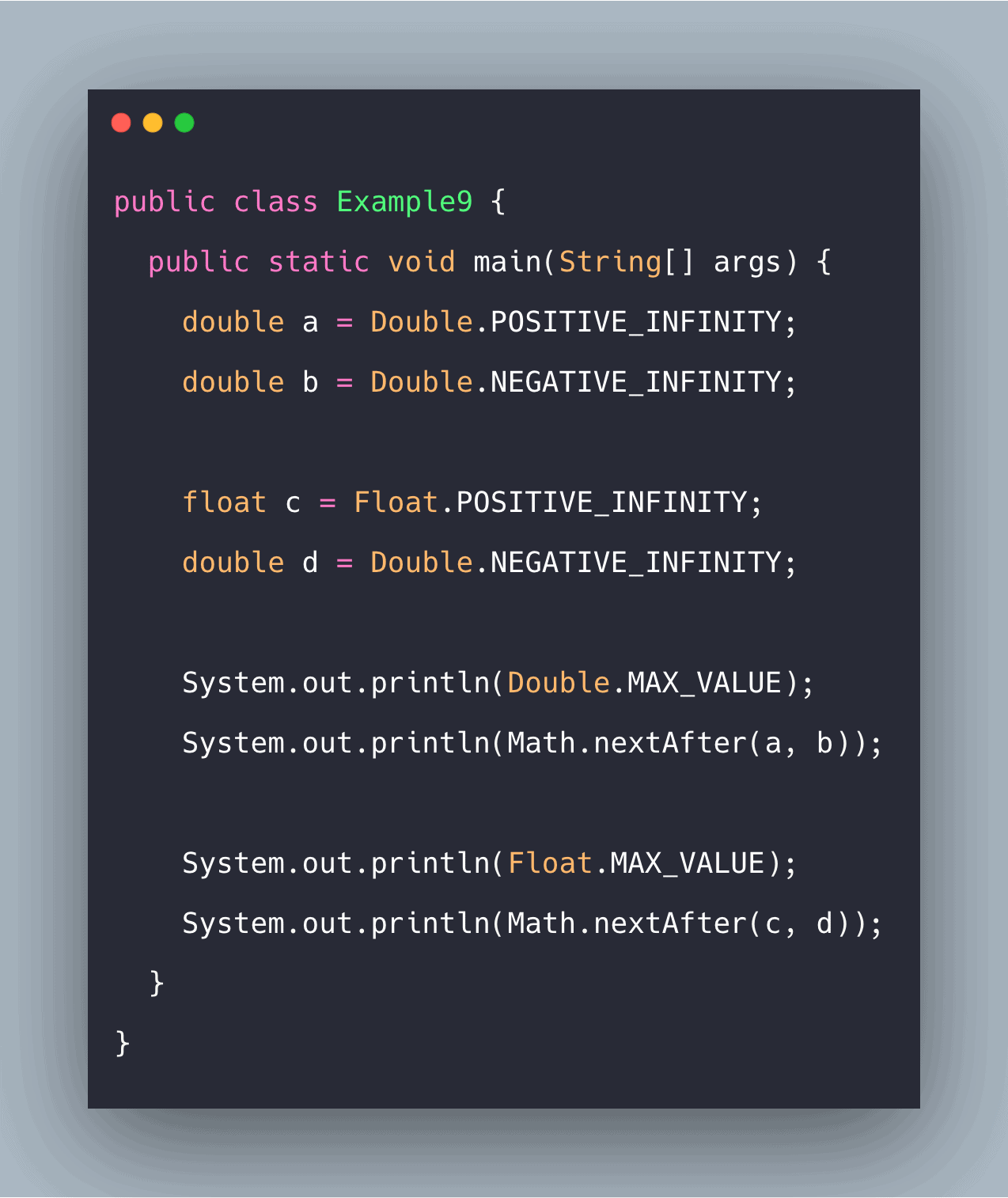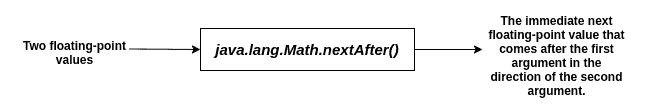# Java Math nextAfter() Function: Complete Guide

0
1Java nextAfter() method comes in handy because floating-point numbers are not equidistant and are finite in number. It is to be noted that a nextAfter implementation of the equivalent nextUp or nextDown implementation may run slower. The method is under the Java Math class.

## Java Math nextAfter()

The java.lang.Math.nextAfter() is a built-in method used to find the immediate next floating-point value that exists after the first argument towards the direction of the second argument. Java Math.nextAfter() method returns the floating-point number adjacent to the first argument in the direction of the second argument.

### Syntax

```public static float nextAfter(float a, double b)
public static double nextAfter(double a, double b)
```

### Parameter(s)

Two floating-point values.

### Return Value

The immediate next floating-point value that exists after the first argument towards the direction of the second argument.#### Note:

• If the arguments are equal, the same floating-point value is returned.
• If one of the arguments is NaN, then this method returns NaN.
• If the first argument is Double.MIN_VALUE (or Float.MIN_VALUE) and the second argument requires a smaller value to be returned, and this method returns a zero with the same sign as the first argument.
• If the first argument is Double.MAX_VALUE (or Float.MAX_VALUE) and the second argument requires a larger value to be returned, and this method returns infinity with the same sign as the first argument.
• If the first argument is infinite and the second argument requires a smaller value to be returned, this method returns Double.MAX_VALUE (or Float.MAX_VALUE) with the same sign as the first argument.
• nextAfter(d, Double.POSITIVE_INFINITY) [or nextAfter(f, Float.POSITIVE_INFINITY)] and nextUp are semantically equivalent.
• nextAfter(d, Double.NEGATIVE_INFINITY) [or nextAfter(f, Float.NEGATIVE_INFINITY)] and nextDown are semantically equivalent.

Consider the following examples.

Example1.java: The following example demonstrates the use of this method.

```public class Example1 {
public static void main(String[] args) {
double a = 14.6;
double b = 45.22;

float c = 12.6f;
double d = 1.2;

System.out.println(Math.nextAfter(a, b));
System.out.println(Math.nextAfter(c, d));
}
}
```

#### Output

```javac Example1.java
->java Example1
14.600000000000001
12.599999
```

#### Example2.java: The following example demonstrates the use of this method on negative floating-point values.

See the following code.

```public class Example2 {
public static void main(String[] args) {
double a = -14.6;
double b = -45.22;

float c = -12.6f;
double d = -1.2;

System.out.println(Math.nextAfter(a, b));
System.out.println(Math.nextAfter(c, d));
}
}
```

#### Output

```->javac Example2.java
->java Example2
-14.600000000000001
-12.599999
```

#### Example3.java: The following example demonstrates the relation between nextUp and nextAfter methods.

See the code.

```public class Example3 {
public static void main(String[] args) {
float a = 104.451f;
double b = 736.147;

System.out.println(Math.nextUp(a));
System.out.println(Math.nextAfter(a, Float.POSITIVE_INFINITY));

System.out.println(Math.nextUp(b));
System.out.println(Math.nextAfter(b, Double.POSITIVE_INFINITY));
}

}
```

#### Output

```->javac Example3.java
->java Example3
104.451004
104.451004
736.1470000000002
736.1470000000002
```

#### Example4.java: The following example demonstrates the relation between nextDown and nextAfter methods.

See the following code.

```public class Example4 {
public static void main(String[] args) {
float a = 104.451f;
double b = 736.147;

System.out.println(Math.nextDown(a));
System.out.println(Math.nextAfter(a, Float.NEGATIVE_INFINITY));

System.out.println(Math.nextDown(b));
System.out.println(Math.nextAfter(b, Double.NEGATIVE_INFINITY));
}
}
```

#### Output

```->javac Example4.java
->java Example4
104.45099
104.45099
736.1469999999999
736.1469999999999
```

#### Example5.java: The following example demonstrates the situation of passing equal arguments.

See the following code.

```public class Example5 {
public static void main(String[] args) {
double a = 134.52;
double b = 134.52;

System.out.println(Math.nextAfter(a, b));

}
}
```

#### Output

```->javac Example5.java
->java Example5
134.52
```

#### Example6.java: The following example demonstrates the situation of passing one of the arguments as NaN.

See the following code.

```public class Example6 {
public static void main(String[] args) {
double a = Double.NaN;
double b = 12.4;

float c = 124.1f;
double d = Double.NaN;

System.out.println(Math.nextAfter(a, b));
System.out.println(Math.nextAfter(c, d));
}
}
```

#### Output

```->javac Example6.java
->java Example6
NaN
NaN

```

#### Example7.java: The following example demonstrates the situation of passing the first argument as MIN_VALUE and the second argument requiring to return a smaller value.

See the following code.

```public class Example7 {
public static void main(String[] args) {
double a = Double.MIN_VALUE;
double b = Double.NEGATIVE_INFINITY;

float c = Float.MIN_VALUE;
double d = Double.NEGATIVE_INFINITY;

System.out.println(Math.nextAfter(a, b));
System.out.println(Math.nextAfter(c, d));
}
}
```

#### Output

```->javac Example7.java
->java Example7
0.0
0.0
```

#### Example8.java: The following example demonstrates the situation of passing the first argument as MAX_VALUE and the second argument requiring to return a larger value.

See the following code.

```public class Example8 {
public static void main(String[] args) {
double a = Double.MAX_VALUE;
double b = Double.POSITIVE_INFINITY;

float c = Float.MAX_VALUE;
double d = Double.POSITIVE_INFINITY;

System.out.println(Math.nextAfter(a, b));
System.out.println(Math.nextAfter(c, d));
}
}
```

#### Output

```->javac Example8.java
->java Example8
Infinity
Infinity
```

Example9.java: The following example demonstrates the situation of passing the first argument as infinite and the second argument requiring to return a smaller value.

See the following code.

```public class Example9 {
public static void main(String[] args) {
double a = Double.POSITIVE_INFINITY;
double b = Double.NEGATIVE_INFINITY;

float c = Float.POSITIVE_INFINITY;
double d = Double.NEGATIVE_INFINITY;

System.out.println(Double.MAX_VALUE);
System.out.println(Math.nextAfter(a, b));

System.out.println(Float.MAX_VALUE);
System.out.println(Math.nextAfter(c, d));
}
}
```

#### Output

```->java Example9
1.7976931348623157E308
1.7976931348623157E308
3.4028235E38
3.4028235E38
```

That’s it for this tutorial.

This site uses Akismet to reduce spam. Learn how your comment data is processed.